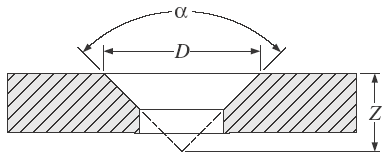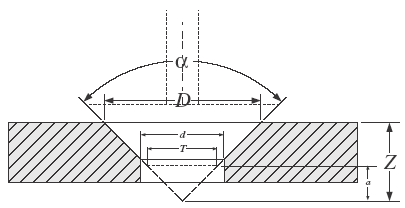Related Resources: calculators

### Countersink and Spot Drill Depth Formula and Calculator

The following formulas are for countersinks and spot drills that have been sharpened to a point, allowances on tool depth will have to be made if the countersink or spot drill is not sharpened to a point.For countersinks and spot drills that have been sharpened to a point

To find the tool travel “Z” required for any sharp-point countersink or spot drill angle, use either of the following formulas:

Z = ( D / 2 ) [ 1 / tan ( α / 2) ]
or
Z = ( D / 2 ) cot ( α / 2)

α = countersink angle
D = countersink diameter
Z = depth of tool travel required from surface of partTo find the tool travel “Z−a” required for any truncated countersink or spot drill angle, use either of the following formulas:

Z - a = ( D - T ) / 2 · [ 1 / tan ( α / 2 ) ]

Z - a = ( D - T ) / 2 · cot ( α / 2 )

a = allowance for truncated countersink tool
d = diameter of through hole
T = diameter of small end of truncated tool

The table below is a quick reference for some predefined To calculate the tool travel “Z”, multiply the factor by the countersink diameter.

 Countersink Angle α, degrees Factor (Imperial or Metric Units) Countersink Angle α, degrees Factor Imperial or Metric Units) 60 0.866 118 0.3004 82 0.5752 120 0.2887 90 0.5000 135 0.2071 100 0.4195 142 0.1722

Related: